•• Merch
• Donate
• SAT Prep

SAT

# 27 Critical SAT Math Concepts to Remember

#### An overview of the main math concepts you should know for the SAT, broken up by how likely you'll be to see them on the test 👏🏼👏🏾👏🏿

So you're a week out from the SAT, and you're realizing that it's probably time to turn off the Netflix and start studying. The full list of topics covered on the SAT Math sections might seem overwhelming, so we've broken it down.

Section 1 below covers 70% of the test. Below that, Section 2 covers another 15% of the test. And below that, Section 3 covers the rest of the test.### Section 1

#### 1. Setting up a linear equation.

You'll need to know how to set up a linear equation given a word problem. There are three things you need to identify: the variable, variable change, and constant. Take a look at this example:Here, our variable is the number of weekly payments, 𝒘. The variable change is the amount associated with 𝒘, which is the weekly cost of $30. And our constant is$60 because it doesn't change each week - it's just the upfront payment. So, the total price of \$300 is equal to the constant plus the added-up weekly payments: 300 = 30𝑤 + 60.

Still unsure about how to handle linear equations? Check out our linear equations lesson for more help.

#### 2. Graphs of linear equations.

You'll also need to know how to define a linear equation from a graph and how to graph a linear equation. Here's an example: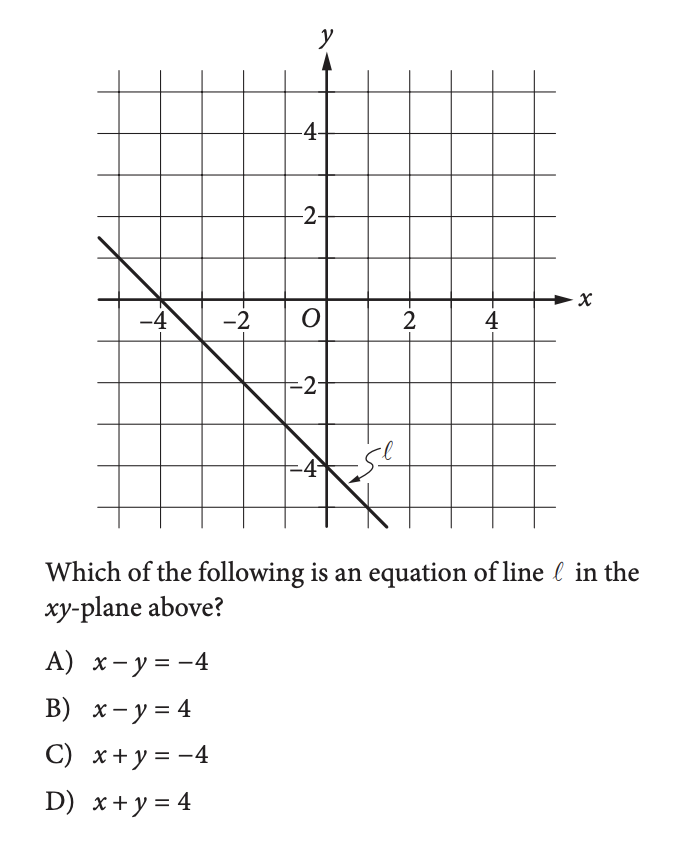Based on the graph, you need to figure out the equation of the line. We do this by identifying the slope and the y-intercept. The y-intercept is the easiest one - it's the value of y the line crosses the vertical y-axis at. In this case, it's -4.

The slope is the change in y divided by the change in 𝑥 between two points (if you're not sure what that means, go through our slope lesson). In this case, it's -1. Our line is then y = -𝔁 - 4, which is equivalent to Choice C.

If that was confusing for you, check out our slope-intercept lesson.

#### 3. Solving a 1-variable equation.

Given an equation with just one variable, you should be able to solve for that variable. For example: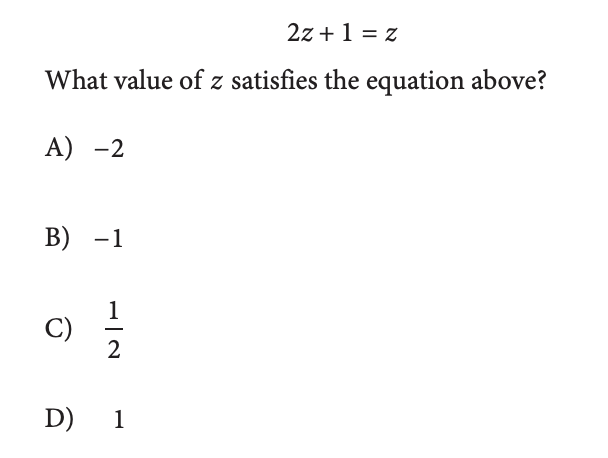Here, we have one variable, 𝒛, that we need to solve for. By grouping all the 𝒛's together, we find that 𝒛 = -1. If you're not sure how to do this, check out linear equations lesson.

#### 4. Plug and Chug

There will be some questions that will ask you which values for a variable make an equation true. Sometimes, it will be easiest to solve the equation, but other times it's easier to just plug in values and test to see what works.

One sign that you should just plug in values is if the equation includes exponents or square roots, like this one: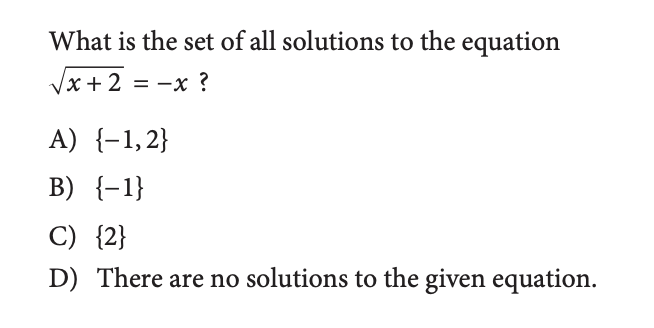Because the negatives can get confusing, it's safest to plug in the values and see what works. The values we need to test are 𝑥=-1 and 𝑥=2. Once we plug them in, we find that the only one that works is 𝑥=-1.

#### 5. Ratios & Proportions

You'll need to know how to set up ratios and how to cross-multiply. The problems might ask you to convert from one unit to another or to figure out the value of something based on a ratio you're given. For example -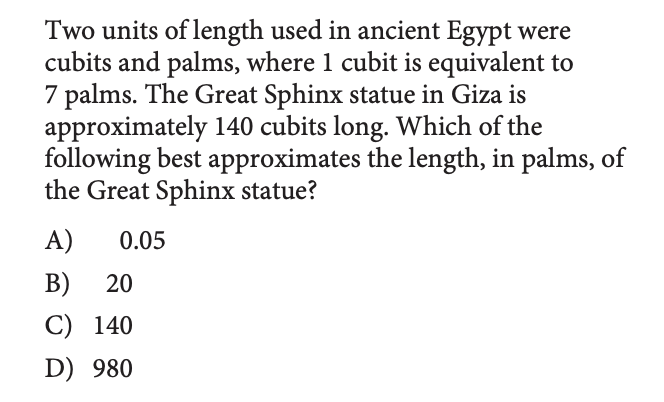In this problem, we're told that 1 cubit = 7 palms. Then we're asked, basically, how many palms are in 140 cubits. We need to know how to set up our ratios into this proportion:If you're not familiar with setting up proportions and cross-multiplying to solve for an unknown variable, check out this lesson.

#### 6. Systems of Linear Equations

You'll need to know how to solve a system of linear equations and what solutions look like on a graph (a solution is where they intersect on the graph).

One thing you should always check for systems of linear equations is whether you can super easily solve by combining the two equations. Like in this example: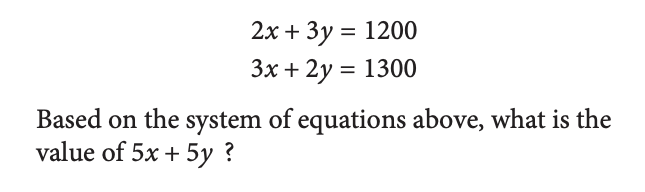By just adding the two equations, we end up with 5𝑥+5y = 2500, so our answer is 2500.

#### 7. Infinite vs. No Solution

The key thing with systems of linear equations is to know what it means to have infinite solutions or no solutions.

An equation can only have infinite solutions if the two equations are exactly equal. And an equation has no solutions if the two lines are parallel, which means they have the same slope, but different y-intercepts. So, when you have a question like this: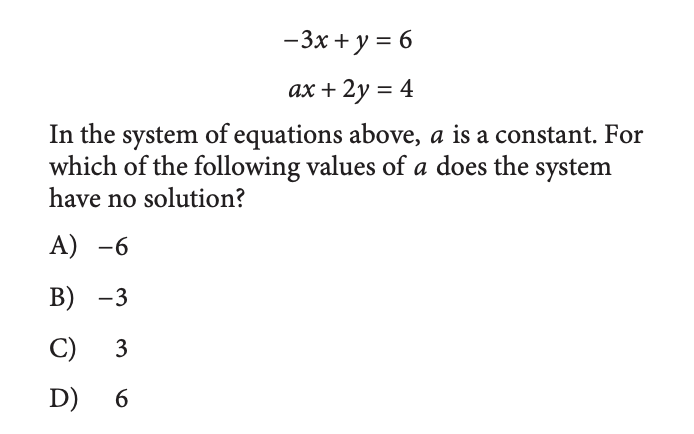We need 𝑎 to be a value that gives the two equations the same slope. Written in slope-intercept form, our first equation is y=3𝑥+6. So, we need the second equation to also have a slope of 3. Written in slope-intercept form, our second equation is:The only value for 𝑎 that will give us a slope of 3 is 𝒂 = -6.

#### 8. Mean, Median, Mode, Standard Deviation, Range

You'll need to remember how to compute each of these, and we'll show you using an example set of numbers: {7, 7, 8, 10, 13}

• Mean = the average of a set of numbers. The mean of our example set is (7+7+8+10+13)/5 = 9.

• Median = the middle number when the numbers are in order from smallest to largest. The median of our example set is 8.

• Mode = the number that shows up the most in a set of numbers. The mode of our example set is 7.

• Range = the biggest number minus the smallest number in a set. The range of our example set is 6.

• Standard Deviation = how spread out the numbers are. You don't need to know how to calculate it, you just need to know that our example set has a bigger standard deviation than a set like {7, 7, 7, 7, 7}.

Some of the questions will just ask you to calculate these values, others will require you to really understand what they mean. Like this one: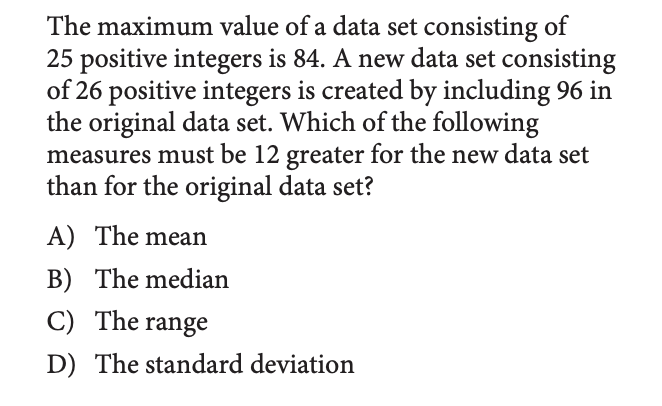We don't have enough information to calculate each of the choices and see which one is 12 greater. But, we DO know that the range is the difference between the biggest number and the smallest number. In this case, the biggest number is increasing from 84 to 96, which is by exactly 12. So, the range must be 12 greater in the new data set.

#### 9. Percent Increase / Decrease

You'll need to know how to set up an equation to calculate the value of something after a percent increase or decrease. This is just generally important in life, so you know what a 60% discount actually is. Take a look at this example: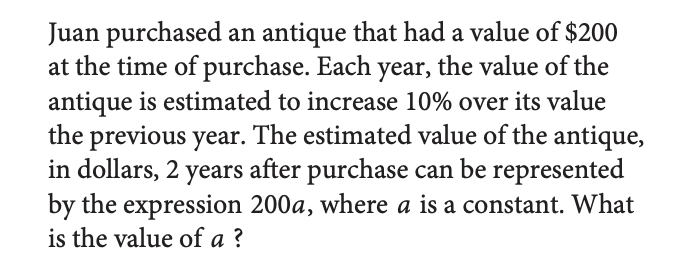When the value of something increases by 10%, we multiply the value by 1 + 0.10 = 1.10. If the value were to decrease by 10%, we would multiply the value by 1 - 0.10 = 0.90.

In this case, we multiply the initial value of 200 by 1.10 after the first year to get 200(1.10) then multiply it by 1.10 again for the second year to get 200(1.10)(1.10). This makes 𝑎 = (1.10)(1.10) = 1.21.

#### 10. Angles

Here are the rules you need to remember for angle calculations:

• A right angle is equal to 90 degrees and is represented by a small square.

• The sum of the angles on a straight line is equal to 180 degrees.

• The sum of the angles around a circle is equal to 360 degrees.

• The sum of the angles in a shape with 𝑛 sides is equal to 180(𝑛 - 2).

• Vertical and parallel angles are equal. In the diagram below, green and blue are vertical angles. Green and purple are parallel angles and blue and orange are also parallel.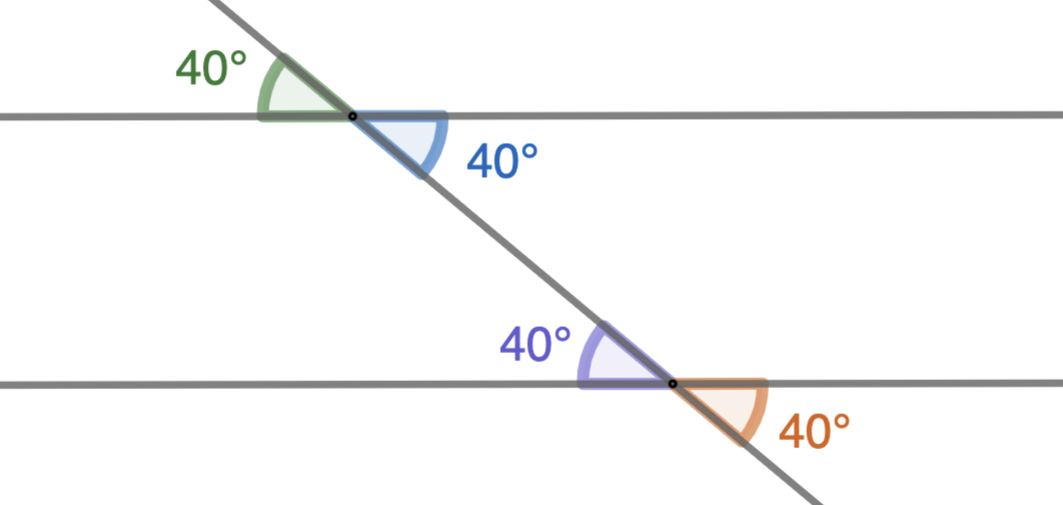The idea is to gradually fill in the angles that you know until you get to the angle in the question. So, take this example question: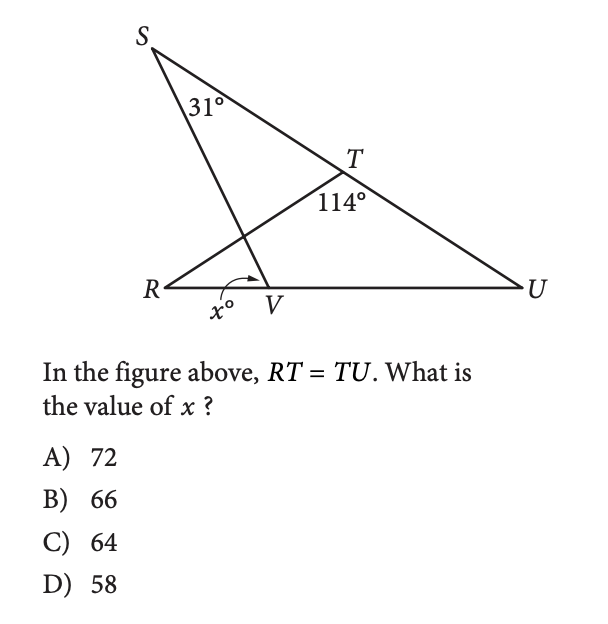And here's what happens when we start filling in angles: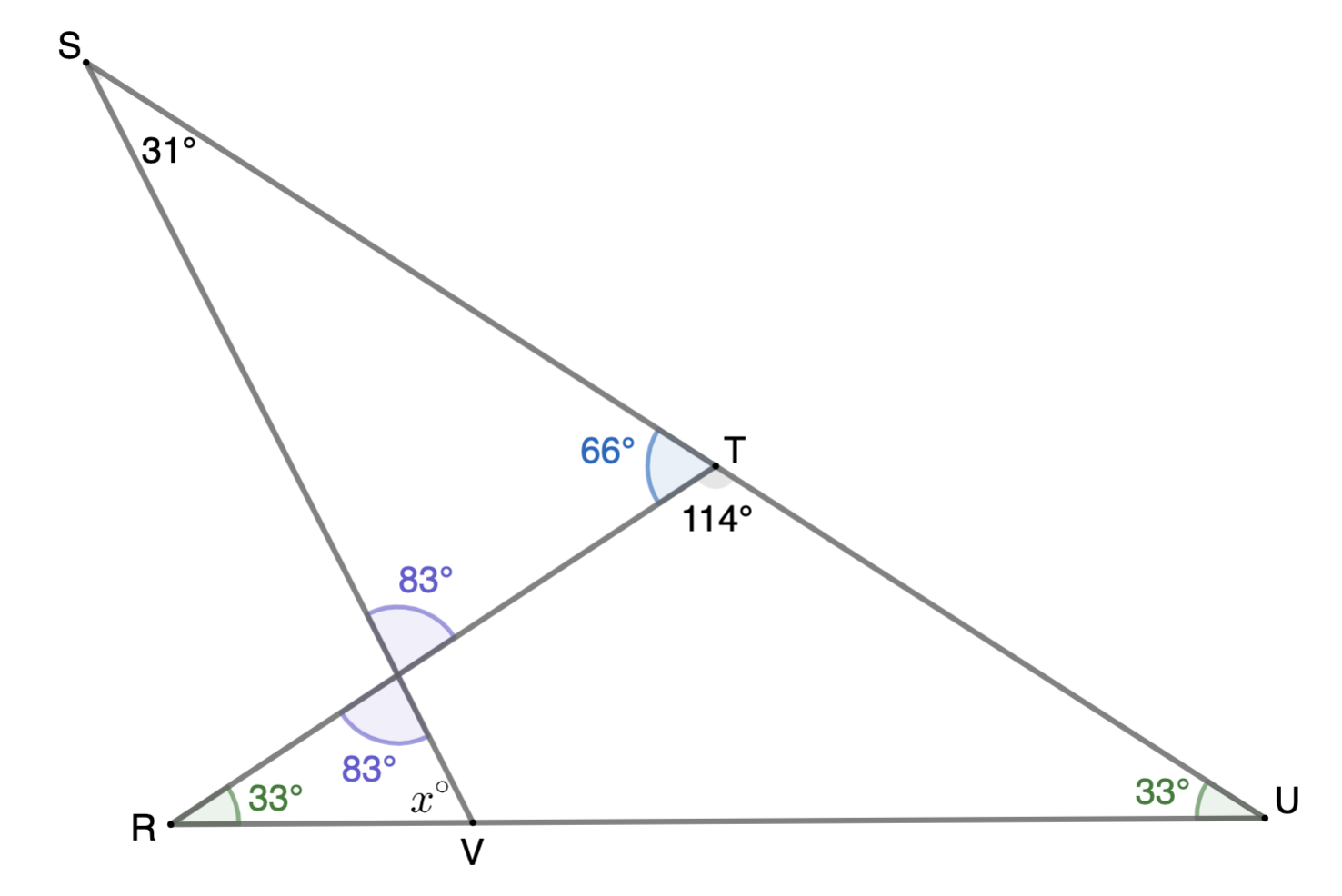The question tells us that RT = TU, so we know the two green angles are equal, and since they're part of a triangle with angle T, we know they're each 33 degrees. Then, we know the blue angle and angle T are equal to 180 degrees because they're on the same line. So, the blue angle is equal to 66 degrees. Then, we can figure out the purple angle is 83 degrees because the sum of the purple angle, blue angle, and S angle is equal to 180 degrees.

Then, we know that the other purple angle is also 83 degrees because they're vertical angles. Finally, the purple angle, green angle, and 𝑥 angle are equal to 180 degrees, so we know the 𝑥 angle is 64 degrees. Done!

You'll need to know how to factor and solve a quadratic equation and how to graph a quadratic equation. Take a look at this example: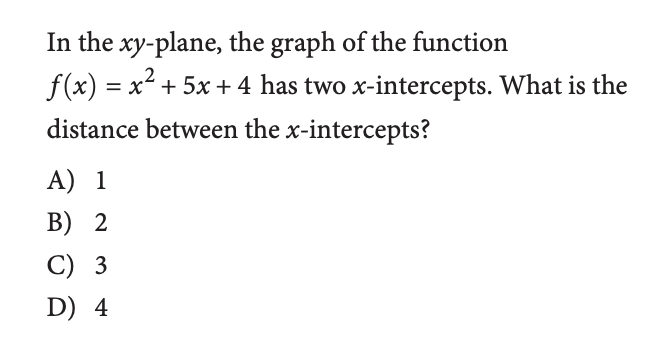First, we need to factor the function, which means we want it in the form of (𝑥 + __)(𝑥 + __). To do this, we need to find two numbers whose sum is 5 and product is 4. Those two numbers are 4 and 1. So, we can factor the function into (𝑥 + 4)(𝑥 + 1). This means that when we graph the function, the 𝑥-intercepts are at -4 and -1.

You can also use the quadratic formula to solve for roots. If your equation is in the formThen, we can solve for our roots with this formula:#### 12. Simplify Polynomials

You'll need to know how to distribute, multiply, divide, and simplify polynomials, which are expressions with variables and exponents. So let's remember the rules:

• We can multiply the same variable or number with different exponents, and we do it by adding the exponents. So, 𝑥⁵(𝑥⁴) = 𝑥⁹.

• We can divide the same variable or number with different exponents, and we do it by subtracting the exponents. So, 𝑥⁵÷𝑥⁴ = 𝑥¹.

• We can distribute by multiplying: 𝑥⁵(𝑥⁴ + 3) = 𝑥⁹ + 3𝑥⁵.

• We cannot add variables or numbers with the same exponents. So, we cannot simplify 𝑥⁵ + 𝑥⁴ any further.

• We cannot subtract variables or numbers with different exponents. So, we cannot simplify 𝑥⁵ - 𝑥⁴ any further.

• To distribute an exponent, we have to multiply all the pairs. So, we distribute (𝑥 + 3)² as (𝑥 + 3)(𝑥 + 3) = 𝑥² + 6x + 9.

Whew. Good work! Remember, if you master Section 1, you'll be covering 70% of the test already. If you're up for more, keep reading below to cover another 15% of the test. But first - a snack break.### Section 2

#### 13. Functions

You'll need to know how to plug something into a function to get an output, how to graph a function, and how to read the graph of a function. If you're not sure what a function is, check out our functions lesson.

So, whether it's just plugging in input values to get an output value (the answer here is 1):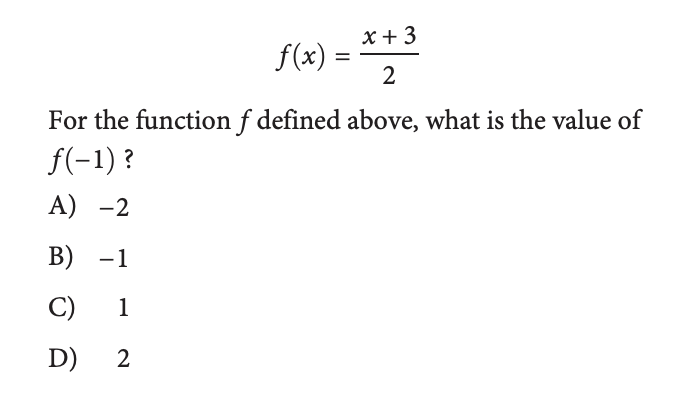Or reading the graph of a function (the answer here is 4):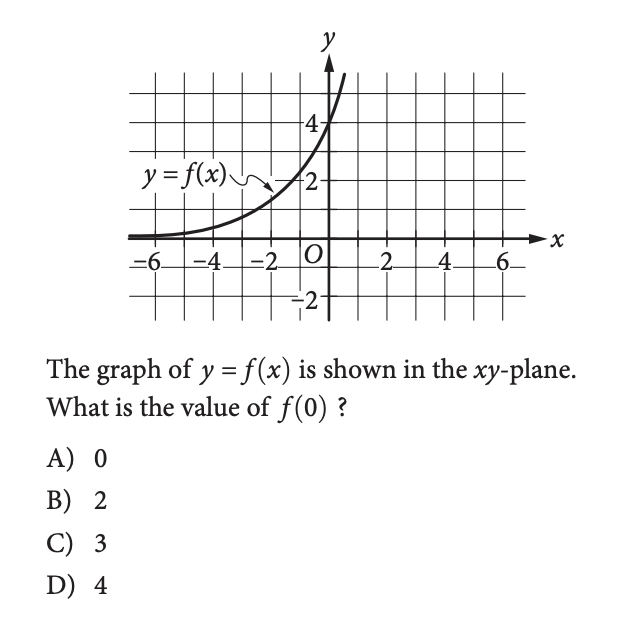You need to have a good understanding of what a function is and how to use it.

#### 14. Function Operations

You'll also need to know how to work with more than one function. This means adding, subtracting, dividing, or multiplying two functions. Like this question, for example: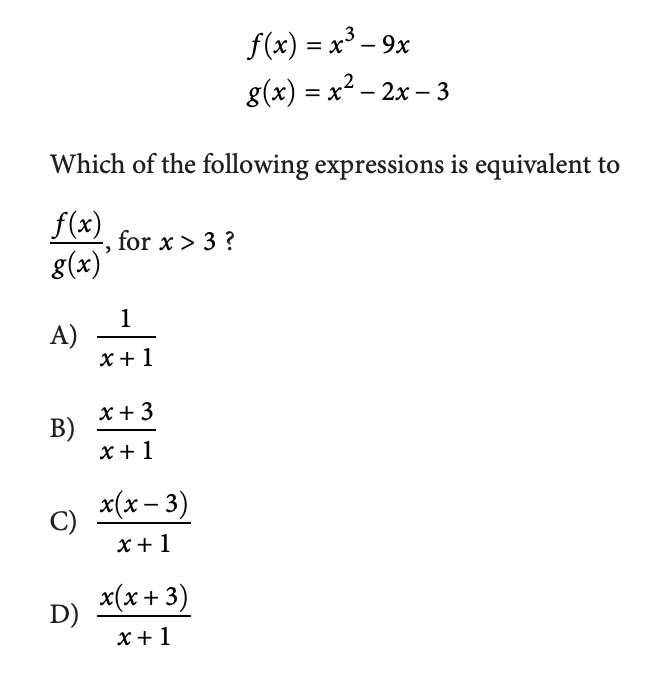We treat this like any other division problem: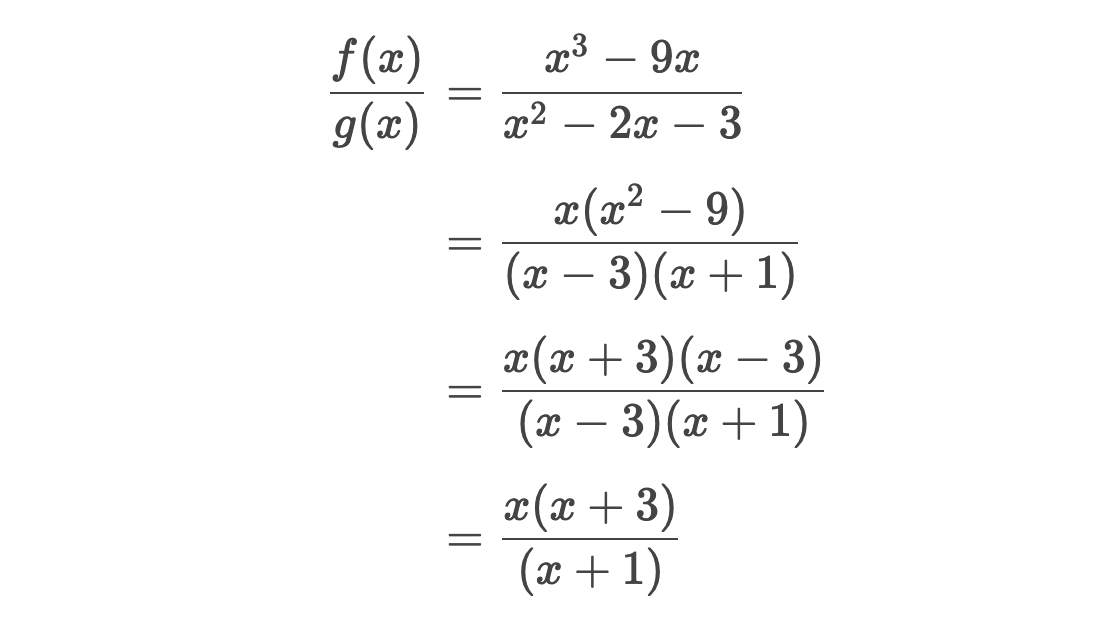Similarly, you would treat function addition and subtraction like any other addition or subtraction problem.

#### 15. Sampling

You'll need to know that a random sample is a small group within a larger group of people that is representative of that larger group. So, for example, a group of 10 seniors would not be a random sample of the students at a high school. But, a group of 10 randomly selected students across all grades is a random sample of the students at a high school.

When you have data from a random sample, remember that you can use it to estimate values for the larger group. Like in this problem: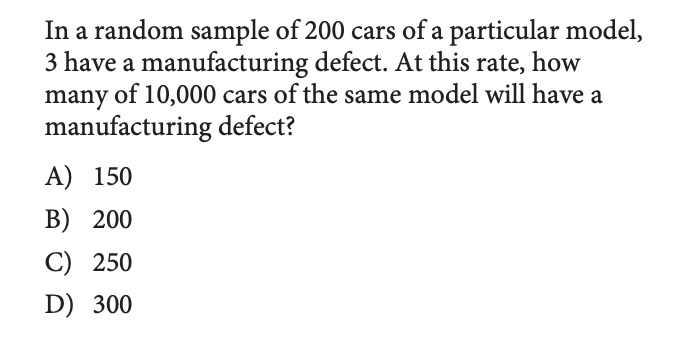Here, we know that 3 out of 200 cars in the sample had a defect. Using this, we can set up this proportion and estimate the number of defective cars in the larger group:You'll also want to remember that margin of error is an estimate of how off your sample is. So, let's say out of sample of 10 of your classmates, 30% think Kanye's only running for president to boost album sales. Then, when we look at the total population of school, we estimate that the margin of error is about 5%. That means that within the total population of your school, the percent that think Kanye's only running to boost sales is somewhere between 30% - 5% = 25% and 30% + 5% = 35%.

Like in this example: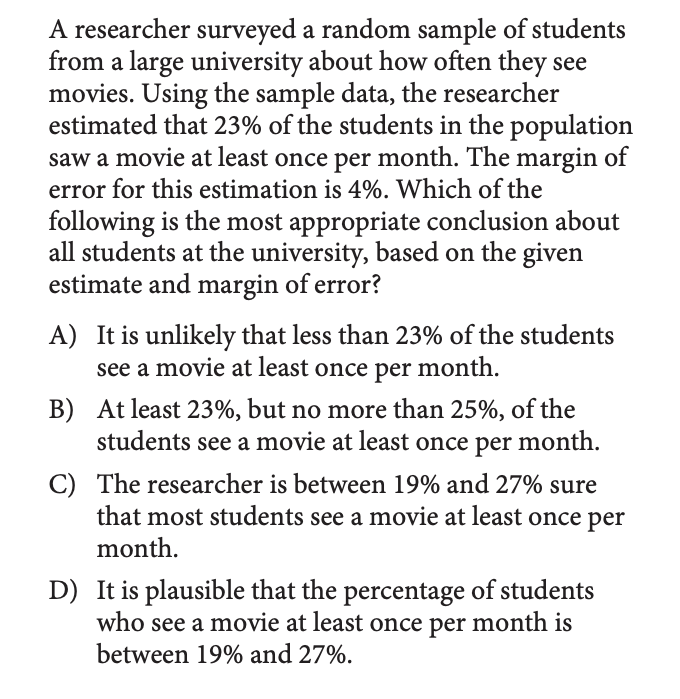From the problem, by understanding sampling and margin of error, we know that the percentage of students who see a movie at least once per month should be between 23% - 4% = 19% and 23% + 4% = 27%. This makes our answer Choice D.

#### 16. Probability

Given a chart of different possible events, you need to be able to calculate the probability that one specific event happens. Just remember, probability of an event happening is defined as the number of ways that event can happen divided by the total number of different events that can happen.

So, for example, check out this problem: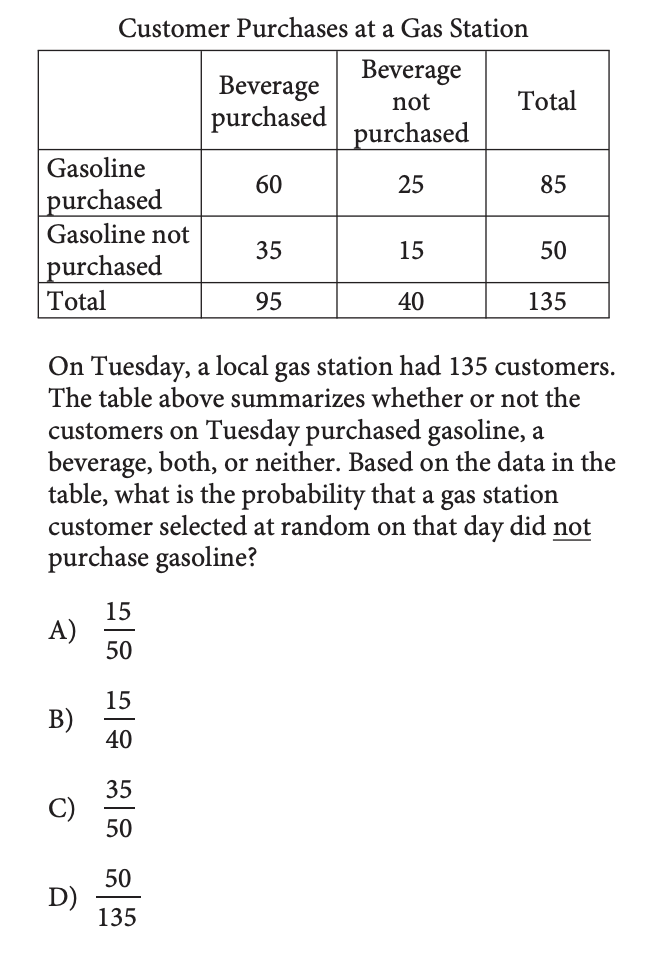We want the probability that a customer at the gas station did not purchase gasoline. From the table, we can see there were 135 total customers at the gas station, and 50 customers (35 + 15) did not purchase gasoline. So, the probability that a customer did not purchase gasoline is 50 / 135, or Choice D.

If we wanted the probability that a customer who purchased a beverage did not purchase gasoline, it would be 35 / 95, because the total would change to the number of people who purchased a beverage.

#### 17. Circles

Remember how to find the equation of a circle based on a graph and how to graph a circle based on an equation. Let's look at this example: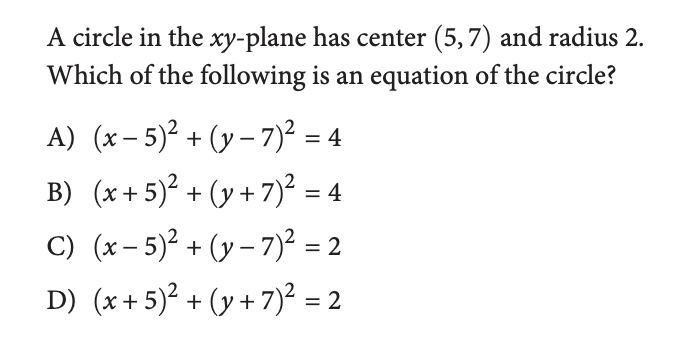Using this example, you'll want to remember that the way you turn a center and a radius into an equation is to negate the center values and square the radius and put them in this form:#### 18. Triangles

First things first, remember these two key facts about triangles:

• There are 180 degrees in a triangle.

• In a right triangle, we can use Pythagorean's theorem to relate the sides of the triangle: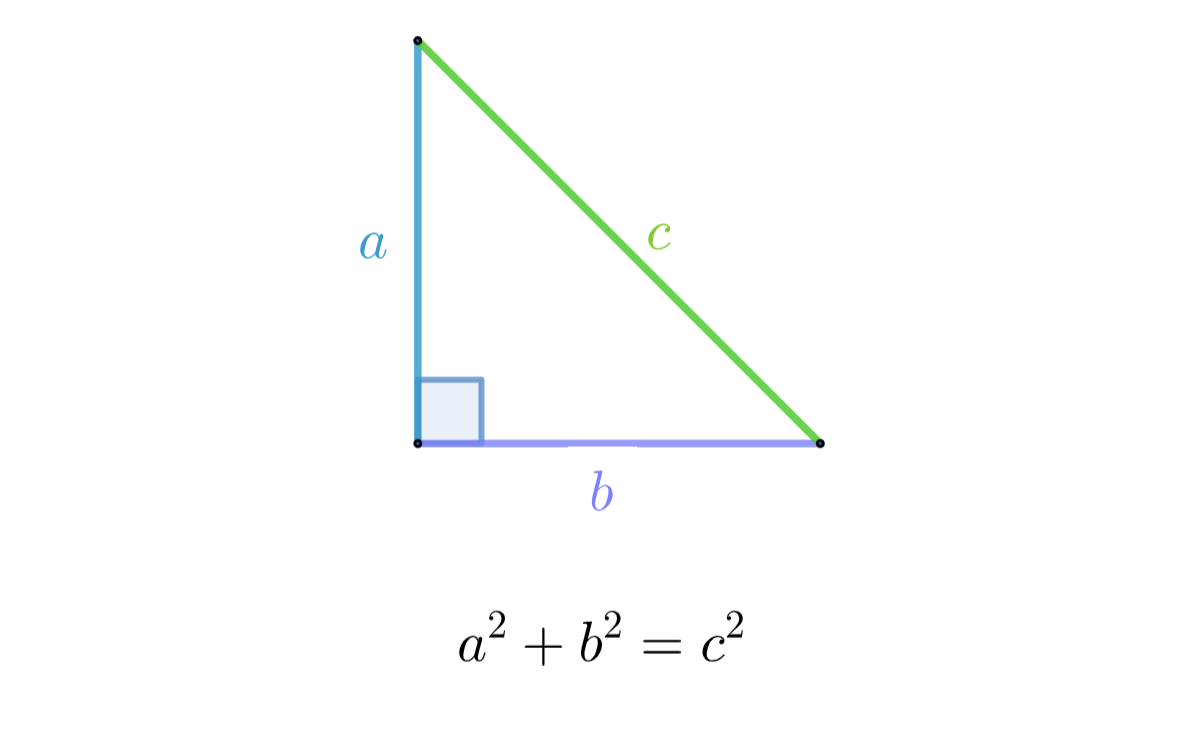On the first sheet of the math section, you'll also see this reference area: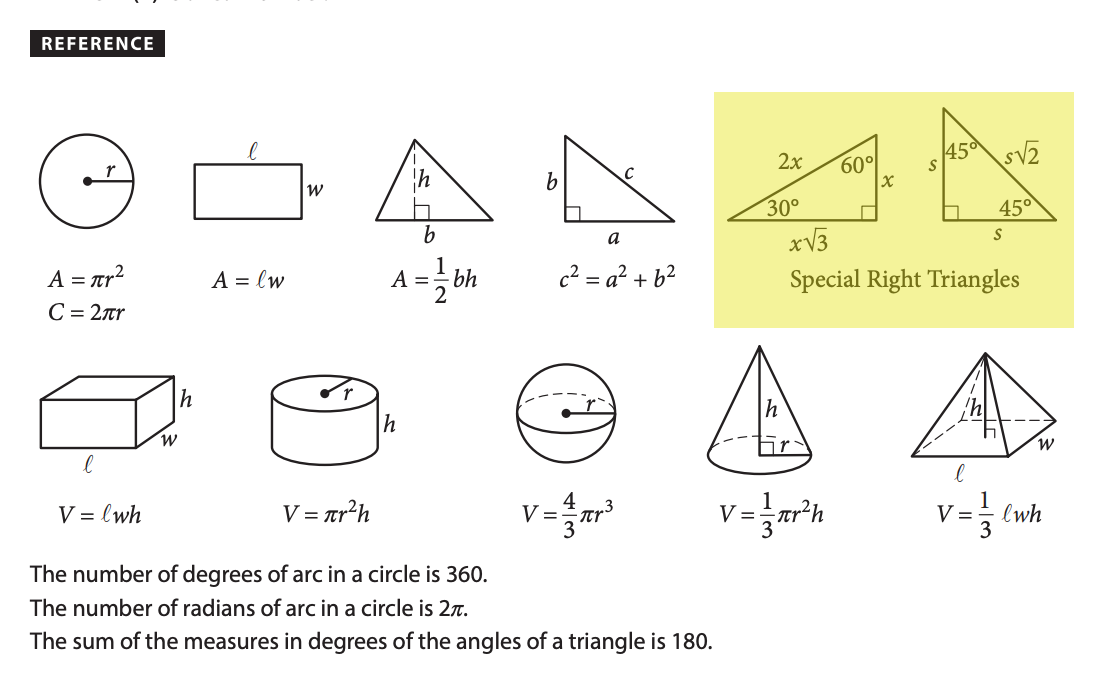Those are two of the special right triangles you should know. You should also know the special right triangle with a ratio of sides 3:4:5. These three special triangles might come up in problems like this: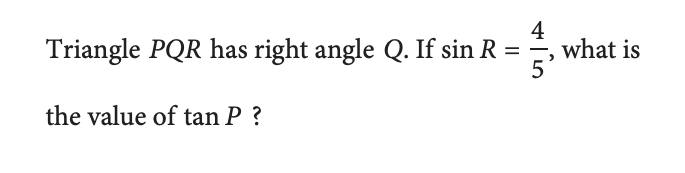By knowing the special 3:4:5 right triangle, we know the other side of this triangle has length 3, which means that tan P is equal to 3/4.

#### 19. Trigonometry

The four important trigonometry facts you should know are:

• Cosine of an angle is equal to the length of the side next to the angle divided by the hypotenuse of the triangle.

• Sine of an angle is equal to the length of side opposite the angle divided by the hypotenuse of the triangle.

• Tangent is equal to sine divided by cosine or the length of the side opposite the angle divided by the length of the side next to the angle.

• cos(𝑥) = sin(90 - 𝑥)

So, take this problem for example: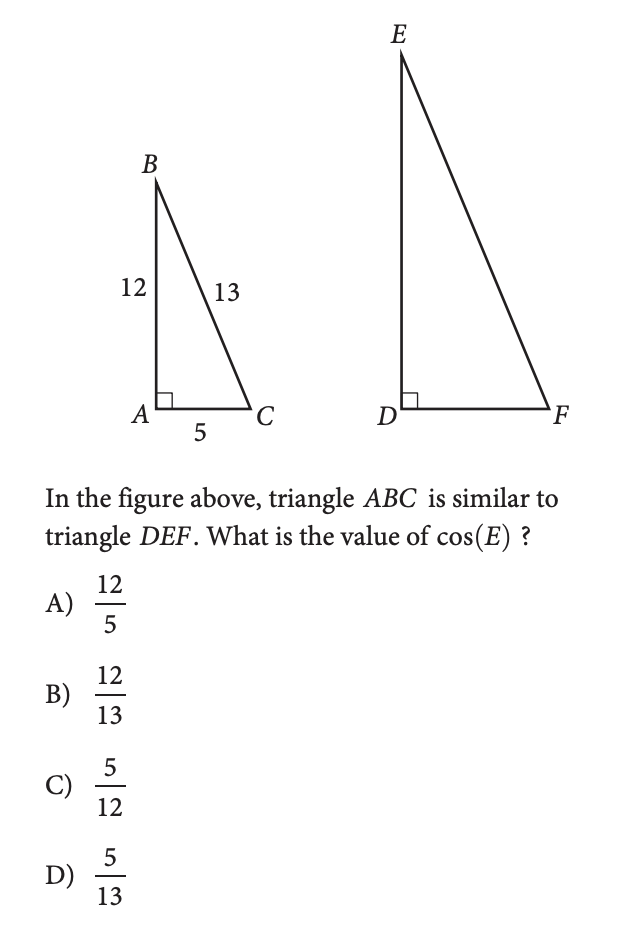Because we know that similar triangles have the same angles, we know that cos(E) = cos(B). And, cos(B) is equal to the length of the side next to B divided by the hypotenuse, which is 12/13, or Choice B.

You should also remember that cos(𝑥) = sin(90 - 𝑥). So, when you get a problem like this: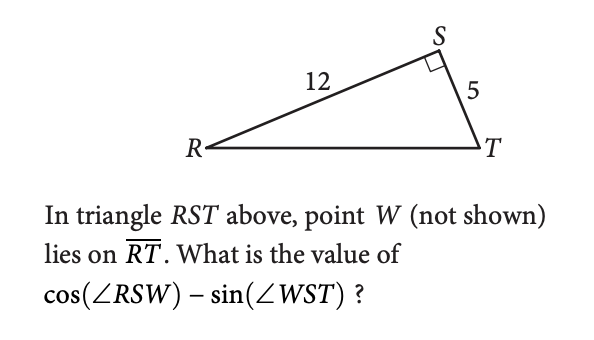You know that the sum of angle RSW and angle WST is 90, which means that cosine of RSW is equal to sine of WST, giving us an answer of 0.

#### 20. Volume

Remember that reference section on the front of the Math section that we mentioned? It also has all the volume formulas you need to know!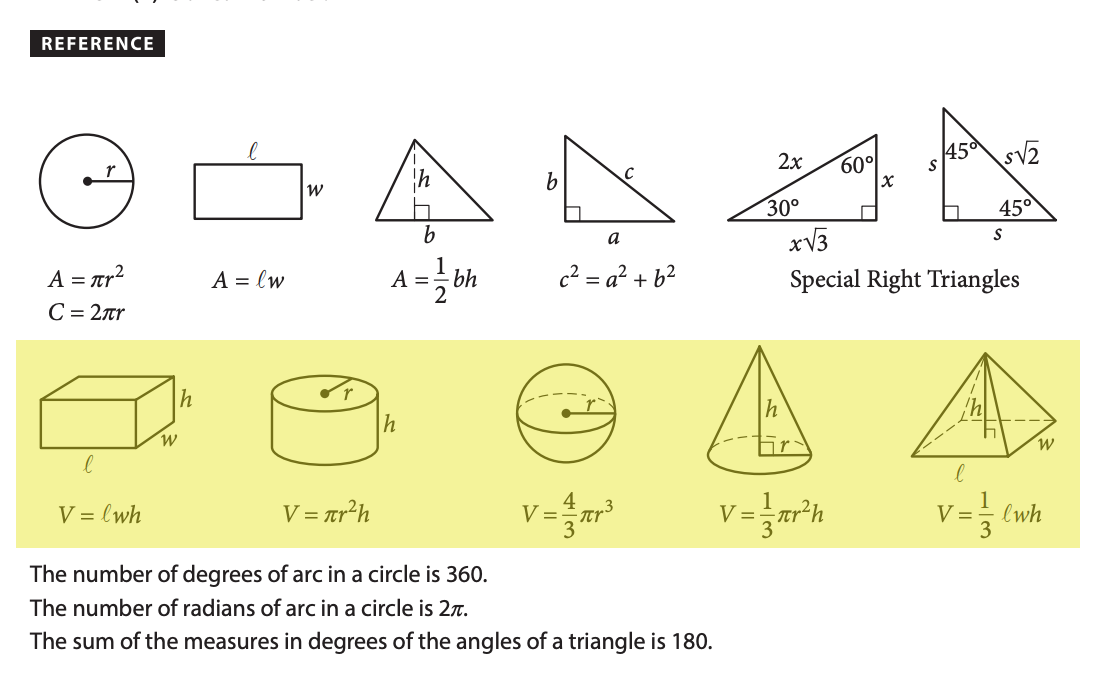We can use these formulas in questions like this one: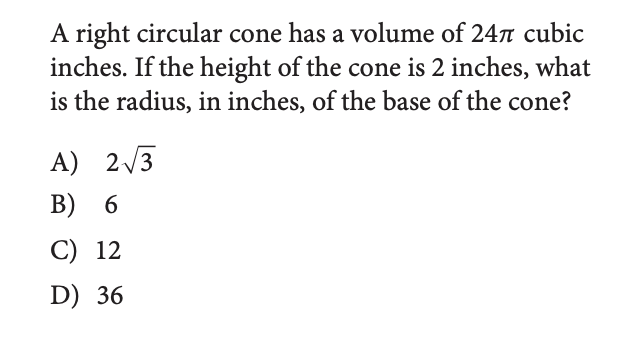If we take a look at our reference table, we can see that the formula for a right circular cone is there, and plugging in values, we get:The one thing you should always make sure to check is that your units are all the same. In this problem, our volume was in cubic inches and our base was in inches, so our radius will also be in inches.

If our base was in feet, we would have needed to convert it into inches before doing our calculation.

#### 21. Inequalities

There are lots of questions with equations on the test, but there are also a few questions on inequalities. Generally, solving an inequality is the same as solving an equation, EXCEPT that when you divide both sides by a negative number, you have to flip the inequality sign.

Mostly, we just want to have a clear understanding of an inequality, so we know how to solve questions like this: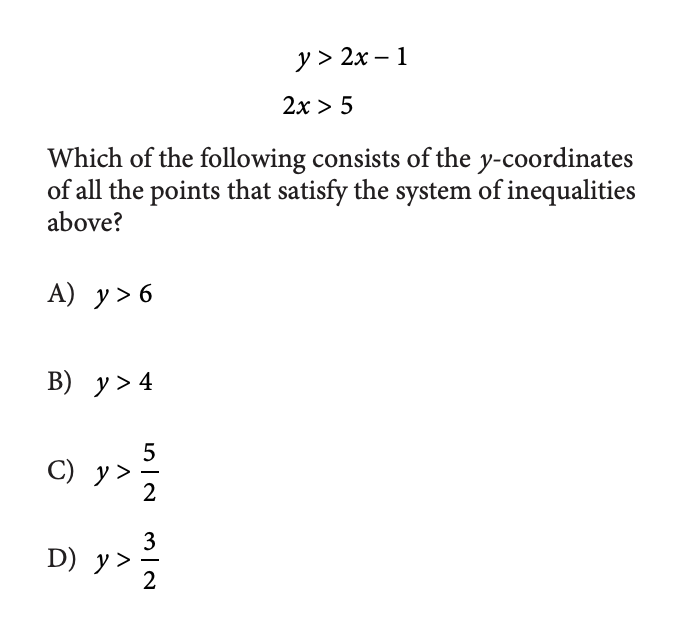From the second inequality, we know that 2𝑥 > 5. So, 2𝑥 cannot be smaller than 5, 5 is the lower bound. Since the first equality is describing the smallest value y can be, we can replace 2𝑥 with it's lower bound of 5.

That means we have y > 5 - 1 which is equal to y > 4.

We also want to know how to graph inequalities. Take a look at this question: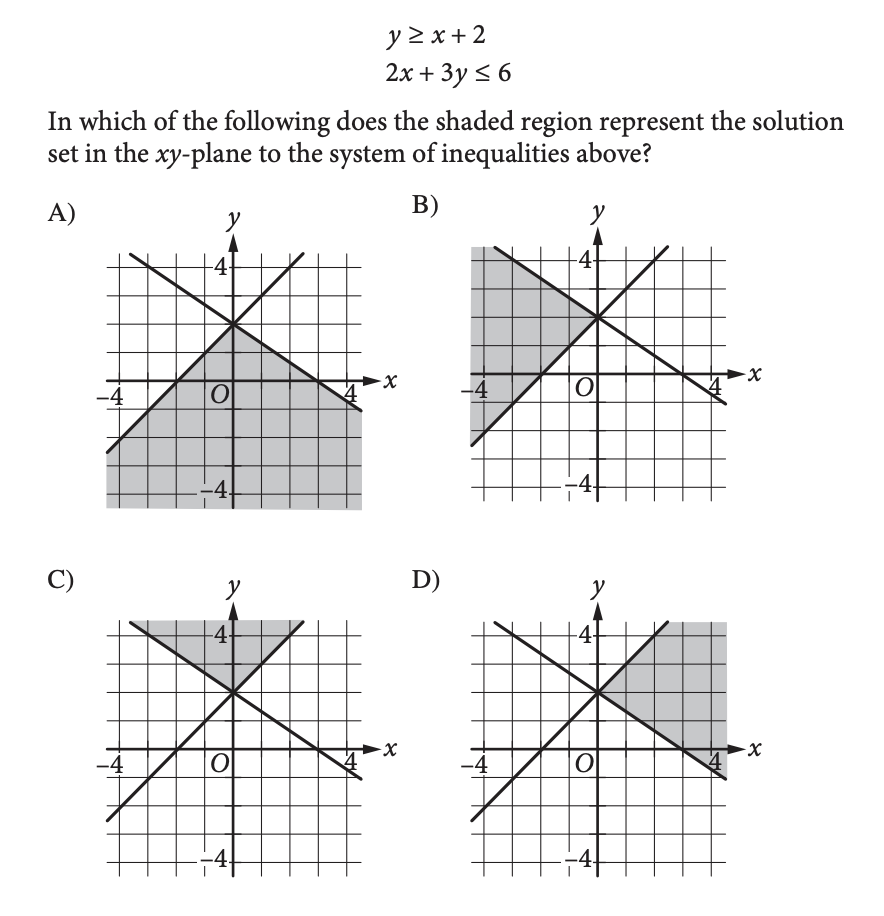The first inequality must be the line going up and to the right since it has a positive slope. And since y is greater than or equal to the line, the shading must be above the line. The other line has y less than or equal to the line, if we rearrange things, so the shading must be below the line. That makes our answer Choice B, which is the overlap area.

Nice! With Sections 1 & 2 covered, we're in good shape for 85% of the test. But if you're still feeling up for it, keep reading to cover the rest of the test. BUT FIRST - take a YouTube break. Trust the process.### Section 3

#### 22. Absolute value.

Absolute value is weirdly not super common on the SAT, but if you're trying to get that perfect score, you should know how to handle them. If you're not super sure about handling absolute value problems, check out our absolute value lesson.

Here's an example absolute value question: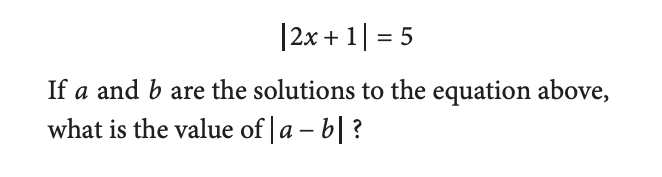This absolute value equation turns into two equations:

• 2𝑥 + 1 = 5

• 2𝑥 + 1 = -5

When we solve each of these we find that 𝑥 = 2 and 𝑥 = -3, which means that our answer is |2 - (-3)| = 5.

The tricky thing about absolute value equations is that, sometimes, you have to plug in your answers in the end and double-check that they were correct. Check out our absolute value equations lesson for more details.

#### 23. Circle Arcs

Basic circle equations are covered in Section 1, but if you want to really own circles, then you'll also want to know how to handle circle arcs. Check out this problem: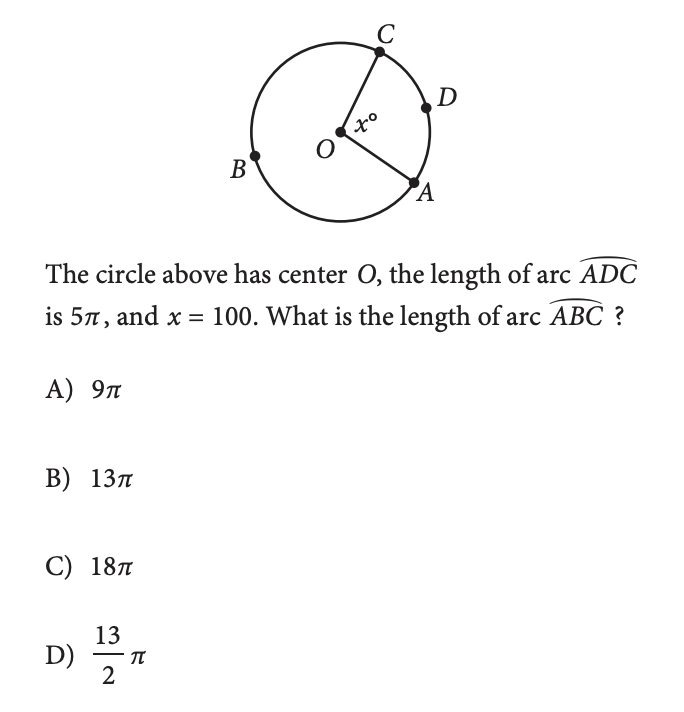See how the angle is in the center of the circle? When this is the case, the ratio of the angle 𝑥 divided by 360 is equal to the arc created by the angle divided by the circumference of the circle. So, this means: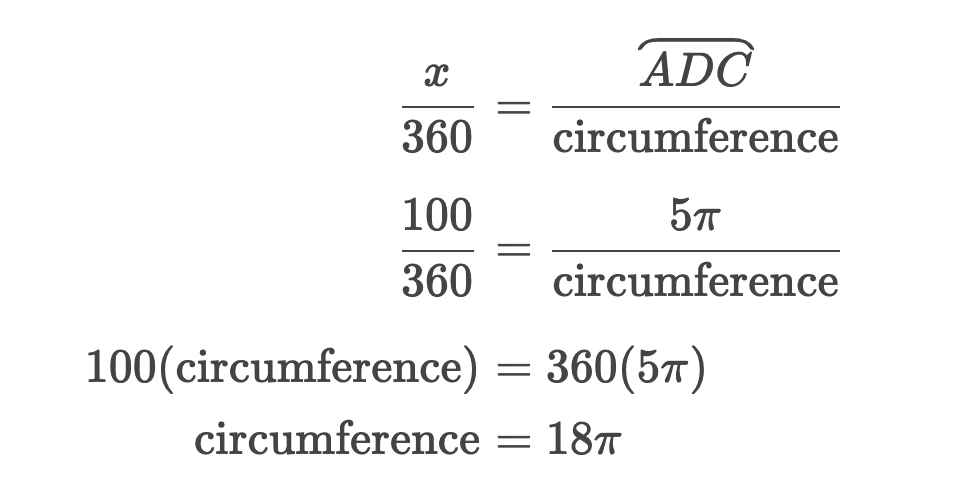If the total circumference is 18𝜋, and arc ADC is 5𝜋, then arc ABC is equal to 18𝜋-5𝜋=13𝜋.

#### 24. Trickier Functions

Basic functions are covered in Section 2, but you'll get a couple harder function questions on the test. With these, we need to have a good understanding of inputs and outputs in functions.

Here's an example question: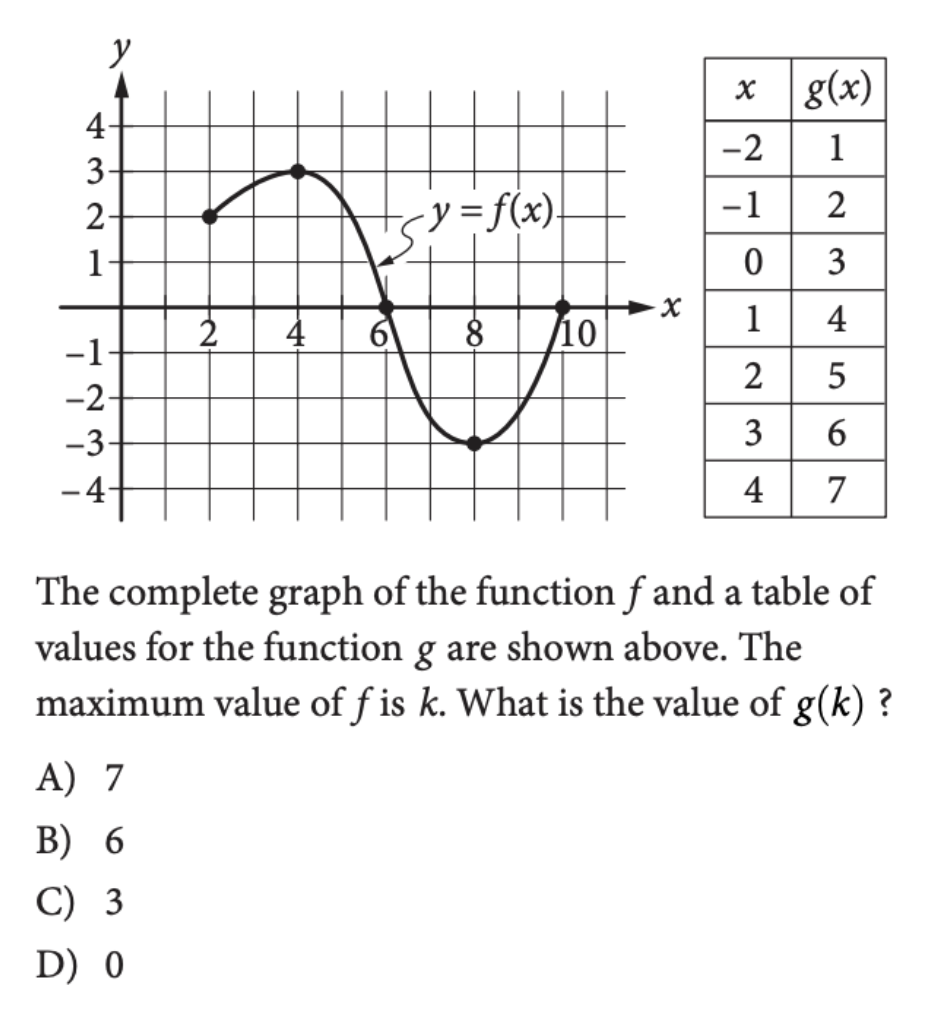First, we need to look at the graph to find the maximum value of f, which is 3. Then, we use this as the input in our function g, which gives us g(3) = 6.

Here's a slightly harder one: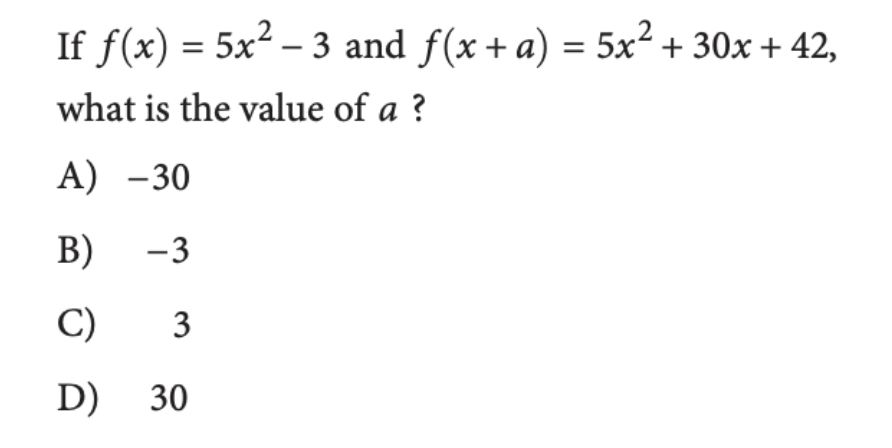We are given the definition of f(𝑥), and then we're given the result when the input plugged in is 𝑥+a. So, let's start by plugging in 𝑥+a into our function and comparing it to the expression given in our problem.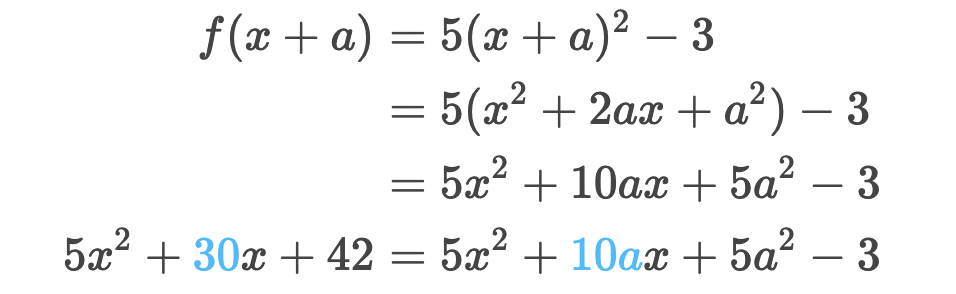When we do this, we see that on both sides, there's only one term with the variable 𝑥 in it, which means the coefficients need to be equal. If 30 = 10a, that means a = 3.

So, just remember, when in doubt, think purely in terms of inputs & outputs. That's all a function is - it's a calculation that gives you an output when you give it an input.

#### 25. Fractional Exponents

Fractional exponents are just like normal exponents, except that they're...fractions. The key thing to remember when you see fractional exponents in an equation is that the way to simplify the equation is to raise everything to the power of the fraction flipped.

So, for example, take a look at this problem: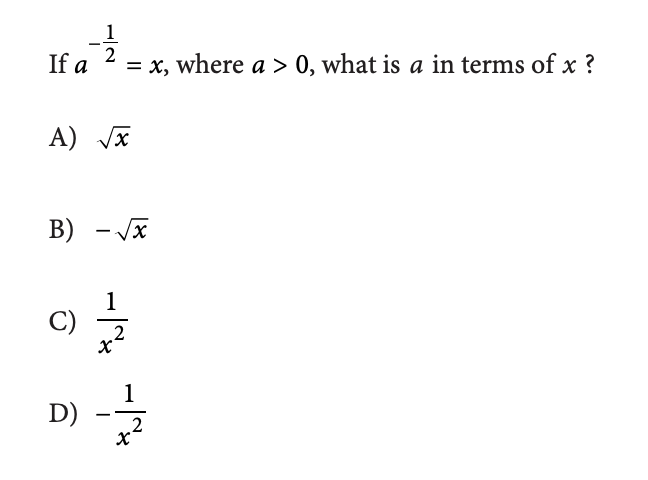We simplify and solve like this: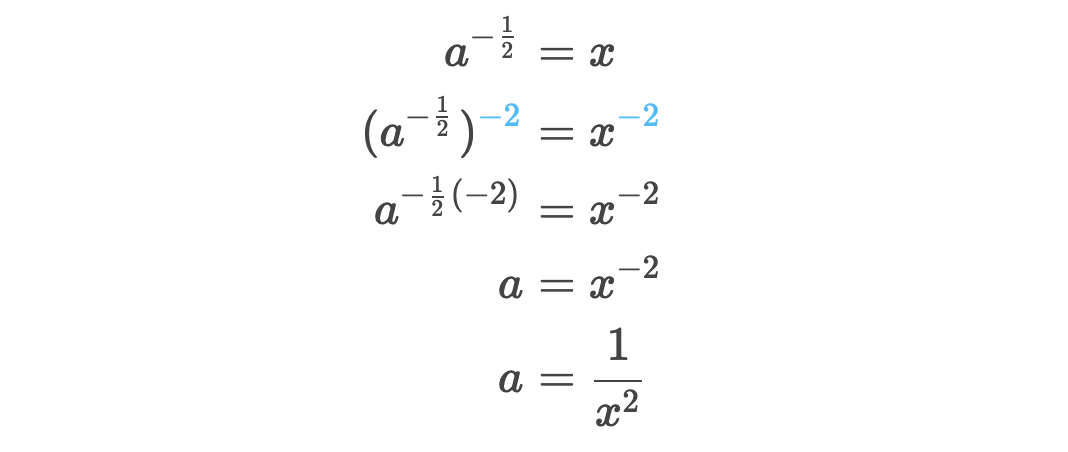See how we raise both sides to the power of -2, which is the inverse of -1/2? That lets us simplify equation and get a on its own. Then, also remember that if a number or variable is raised to a negative power, that's the same as the flipped version of that number or variable raised to the positive version of the power.

#### 26. Graphing Parabolas

When we graph parabolas, we can use the factored form, like y = (x-2)(x+4), to know that the parabola goes through points (2, 0) and (-4, 0).

The other form that we'll want to know is vertex form. This is when the equation looks like this:This form tells us the vertex of the parabola. If a is negative, then the vertex is the highest point of the parabola. If a is positive, then the vertex is the lowest point of the parabola. And from this form, the vertex is (h, k).

Take a look at this example problem: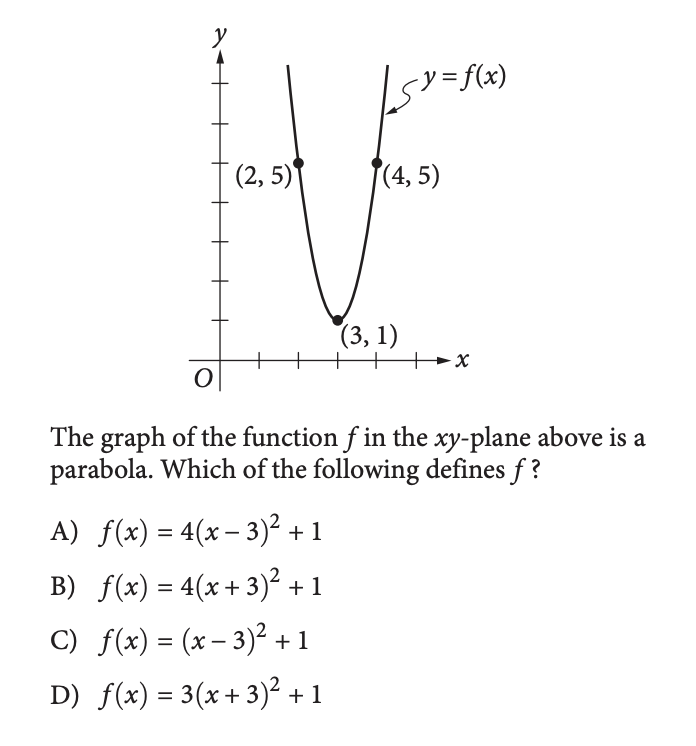From the graph, we can see the vertex is (3, 1). That means the equation of our parabola should look something like this:The only choices that are in this form are Choice A and Choice C, so it must be one of those. When we plug in one of the other points, let's say (4, 5), we can see that Choice A is the only one that gives us that point correctly.

#### 27. Imaginary Numbers

You might get 1-2 imaginary number problems on the test. Imaginary numbers are numbers that are a normal number multiplied by the square root of -1, which we represent as 𝒊. Because we can't really take the square root of a negative number, we call these imaginary numbers.

The cool thing about the square root of -1 is that it cycles as you power it up: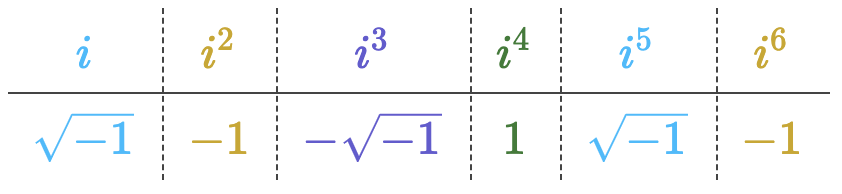The powers of 𝒊 cycle every 4 powers. So, 𝒊 = 𝒊⁵ = 𝒊⁹ = 𝒊¹³ and so on.

Here's an example problem from the test: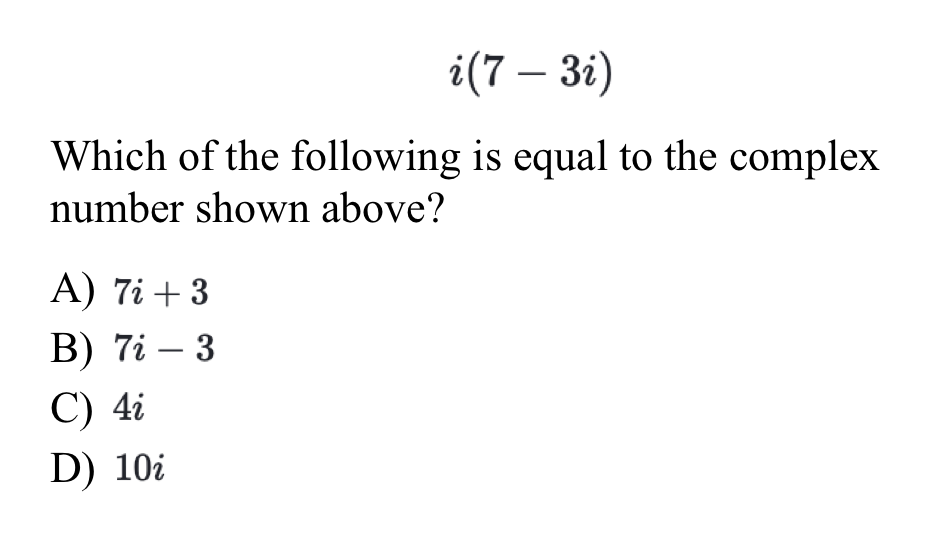When we distribute, we get 𝒊(7-3𝒊) = 7𝒊 - 3𝒊² = 7𝒊 - 3(-1) = 7𝒊 +3.

So, you can distribute and multiply normally - just think of 𝒊 like a variable. But then when you're done simplifying, replace 𝒊 for the square root of -1, and multiply further out.

That's it! That's your guide to the 27 critical math concepts to know for the SAT. Study these and then trust yourself on test day.##### Our Team##### Learning Resources##### Terms & Privacy